# Algebra symbols

List of mathematical algebra symbols and signs.

## Algebra math symbols table

Symbol Symbol Name Meaning / definition Example
x x variable unknown value to find when 2x = 4, then x = 2
= equals sign equality 5 = 2+3
5 is equal to 2+3
5 is not equal to 4
equivalence identical to
equal by definition equal by definition
:= equal by definition equal by definition
~ approximately equal weak approximation 11 ~ 10
approximately equal approximation sin(0.01) ≈ 0.01
proportional to proportional to yx when y = kx, k constant
lemniscate infinity symbol
much less than much less than 1 ≪ 1000000
much greater than much greater than 1000000 ≫ 1
( ) parentheses calculate expression inside first 2 * (3+5) = 16
[ ] brackets calculate expression inside first [(1+2)*(1+5)] = 18
{ } braces set
x floor brackets rounds number to lower integer ⌊4.3⌋= 4
x ceiling brackets rounds number to upper integer ⌈4.3⌉= 5
x! exclamation mark factorial 4! = 1*2*3*4 = 24
| x | vertical bars absolute value | -5 | = 5
f (x) function of x maps values of x to f(x) f (x) = 3x+5
(fg) function composition

(fg) (x) = f (g(x))

f (x)=3x,g(x)=x-1⇒(f g)(x)=3(x-1)
(a,b) open interval (a,b) = {x | a < x < b} x ∈ (2,6)
[a,b] closed interval [a,b] = {x | axb} x ∈ [2,6]
delta change / difference t = t1 - t0
discriminant Δ = b2 - 4ac
sigma summation - sum of all values in range of series xi= x1+x2+...+xn
∑∑ sigma double summation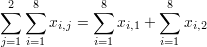capital pi product - product of all values in range of series xi=x1∙x2∙...∙xn
e e constant / Euler's number e = 2.718281828... e = lim (1+1/x)x , x→∞
γ Euler-Mascheroni constant γ = 0.5772156649...
φ golden ratio golden ratio constant
π pi constant π = 3.141592654...

is the ratio between the circumference and diameter of a circle

c = πd = 2⋅πr

## Linear Algebra Symbols

Symbol Symbol Name Meaning / definition Example
· dot scalar product a · b
× cross vector product a × b
AB tensor product tensor product of A and B AB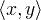inner product
[ ] brackets matrix of numbers
( ) parentheses matrix of numbers
| A | determinant determinant of matrix A
det(A) determinant determinant of matrix A
|| x || double vertical bars norm
A T transpose matrix transpose (AT)ij = (A)ji
A Hermitian matrix matrix conjugate transpose (A)ij = (A)ji
A* Hermitian matrix matrix conjugate transpose (A*)ij = (A)ji
A-1 inverse matrix A A-1 = I
rank(A) matrix rank rank of matrix A rank(A) = 3
dim(U) dimension dimension of matrix A dim(U) = 3

Algebra is a subfield of mathematics pertaining to the manipulation of symbols and their governing rules. The following is a compilation of symbols from the different branches of algebra, which include basic algebra, number theory, linear algebra and abstract algebra.

A mathematical symbol is a figure or a combination of figures that is used to represent a mathematical object, an action on mathematical objects, a relation between mathematical objects, or for structuring the other symbols that occur in a formula. As formulas are entirely constituted with symbols of various types, many symbols are needed for expressing all mathematics.

The most basic symbols are the decimal digits (0, 1, 2, 3, 4, 5, 6, 7, 8, 9), and the letters of the Latin alphabet. The decimal digits are used for representing numbers through the Hindu–Arabic numeral system. Historically, upper-case letters were used for representing points in geometry, and lower-case letters were used for variables and constants. Letters are used for representing many other sort of mathematical objects. As the number of these sorts has dramatically increased in modern mathematics, the Greek alphabet and some Hebrew letters are also used. In mathematical formulas, the standard typeface is italic type for Latin letters and lower-case Greek letters, and upright type for upper case Greek letters. For having more symbols, other typefaces are also used, mainly boldface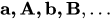script typeface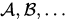(the lower-case script face is rarely used because of the possible confusion with the standard face), German fraktur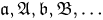and blackboard bold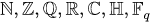(the other letters are rarely used in this face, or their use is unconventional).

The use of Latin and Greek letters as symbols for denoting mathematical objects is not described in this article. For such uses, see Variable (mathematics) and List of mathematical constants. However, some symbols that are described here have the same shape as the letter from which they are derived, such as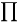and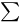.

Letters are not sufficient for the needs of mathematicians, and many other symbols are used. Some take their origin in punctuation marks and diacritics traditionally used in typography. Other, such as + and =, have been specially designed for mathematics, often by deforming some letters, as in the cases of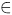and.

Statistical symbols »

Currently, we have around 1975 calculators, conversion tables and usefull online tools and software features for students, teaching and teachers, designers and simply for everyone.